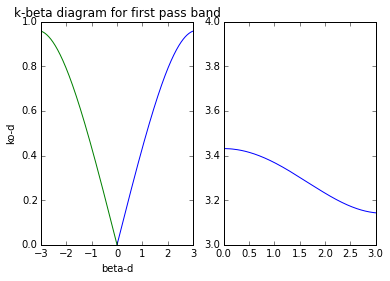# Chapter 8 MICROWAVE FILTERS¶

## Example:8.1 page.no:429¶

In :
# program to compute the propagation constant , phase velocity and bloch impedence .
%matplotlib inline
from pylab import arange,plot,axis,title,xlabel,ylabel,subplot
from numpy import cos,sin,pi,arccos,seterr
from mpmath import acos

Co=2.666*10**-12;
d=0.01;c=3*10**8;
Zo=50.;f=3*10**9;
p=(Co*Zo*c)/(2*d); # constant of equation given below .
y=arange(0,0.96,0.001)
x=arccos(cos(y)-p*y*sin(y)); # x=ko⇤d; and y=beta⇤d;
subplot(121)
plot(x,y)
plot(-x,y)
axis([-3,3,0,1])
title ("k-beta diagram for first pass band ")
xlabel ("beta-d")
ylabel ("ko-d")
y=arange(3,4,0.001)
seterr(all='ignore')
x=arccos(cos(y)-p*y*sin(y)); # x=ko⇤d; and y=beta⇤d ;
subplot(122)
plot(x,y)
axis([0,3,3,4]);## Example:8.2 page.no:441¶

In :
# program to design a low pass composite filter with cutoff frequency of 2 MHZ.
from math import sqrt,pi

fc=2*10**6;f=2.05*10**6;Ro=75;
L=(2*Ro)/(2*pi*fc);
C=2/(Ro*2*pi*fc);
m=sqrt(1-(fc/f)**2)
x=m*L/2;
y=m*C;
z=((1-m**2)/(4*m))*L; # x,y,z are design parameter assumed .
print "design parameter for m=0.2195 ==> %.4e %.4e %.4e \n"%(x,y,z)
m=0.6
x=m*L/2;
y=m*C/2;
z=((1-m**2)/(2*m))*L; # x,y,z are design parameter assumed .
print "design parameter for m=0.6 ==> %.4e %.4e %.4e \n"%(x,y,z)

design parameter for m=0.2195 ==> 1.3101e-06 4.6582e-10 1.2939e-05

design parameter for m=0.6 ==> 3.5810e-06 6.3662e-10 6.3662e-06



## Example:8.3 page.no:449¶

In :
# program to find out number of filter elements required .
from math import pi

fc=8*10**9;f=11*10**9;
w=2*pi*f;
wc=2*pi*fc;
x=abs(w/wc)-1;
print "from table we see that an attenuation of 20 db at this frequency requires that N>=8 for x = ",x

from table we see that an attenuation of 20 db at this frequency requires that N>=8 for x =  0.375


## Example:8.4 page.no:447¶

In :
# program to design a maximum flat low pass filter with cut off frequency of 2 GHZ.
from math import pi

fc=2*10**9;f=3*10**9;
w=2*pi*f;
wc=2*pi*fc;
x=abs(w/wc)-1;
# from table we can see that N=5 will be sufficient .
# then prototype element values are:
g1 =0.618; g2 =1.618; g3 =2.000; g4 =1.618; g5 =0.618;
print "g1 = ",g1
print "g2 = ",g2
print "g3 = ",g3
print "g4 = ",g4
print "g5 = ",g5

g1 =  0.618
g2 =  1.618
g3 =  2.0
g4 =  1.618
g5 =  0.618


## Example:8.5 page.no:460¶

In :
# design a band pass filter having a 0.5 db equal ripple respnse with N=3.
from math import pi

N=3;Zo=50;f=1*10**9;delta=1*10**8;
L1 =1.596; L3 =1.5963; C2 =1.0967; Rl =1.000;
L_1=(L1*Zo)/(2*pi*f*delta);
C_1=delta/(2*pi*f*L1*Zo);
L_2=(delta*Zo)/(2*pi*f*C2);
C_2=C2/(2*pi*f*delta*Zo);
L_3=(L3*Zo)/(2*pi*f*delta);
C_3=delta/(2*pi*f*L3*Zo);
print "L1- %.2e"%L_1
print "L2- %.2e"%L_2
print "L3- %.2e"%L_3
print "C1- %.2e"%C_1
print "C2- %.2e"%C_2
print "C3- %.2e"%C_3

L1- 1.27e-16
L2- 7.26e-01
L3- 1.27e-16
C1- 1.99e-04
C2- 3.49e-20
C3- 1.99e-04


## Example:8.6 page.no:466¶

In :
# design a low pass filter for fabrication using ￼micrstrip lines .
print "from table , the normalized low pass prototype element values are = "
L1 =3.3487; C2 =0.7117; L3 =3.3487; Rl =1.0000;
n=1+(1/3.3487);
print(L1)
print(Rl)
print(C2)
print(L3)
print "%.3f"%n

from table , the normalized low pass prototype element values are =
3.3487
1.0
0.7117
3.3487
1.299


## Example:8.7 page.no:472¶

In :
# design a steppedimpedence low pass filter having a maximally flat response and a cutoff frequency of 2.5 GHZ.
from math import pi

w=4*10**9;wc=2.5*10**9;Zh=150;Ro=50;Zl=10; 62
C1 =0.517; L2 =1.414; C3 =1.932; L4 =1.932; C5 =1.414; L6 =0.517;
# above values are taken from table.
# for finding electrical lengths .
x1=(C1*Zl/Ro)*(180/pi);
x2=(L2*Ro/Zh)*(180/pi);
x3=(C3*Zl/Ro)*(180/pi);
x4=(L4*Ro/Zh)*(180/pi);
x5=(C5*Zl/Ro)*(180/pi);
x6=(L6*Ro/Zh)*(180/pi);
print(x1)
print(x2)
print(x3)
print(x4)
print(x5)
print(x6)

5.92438360165
27.0054107438
22.1390892039
36.8984820064
16.2032464463
9.87397266942


## Example:8.8 page.no:486¶

In :
# design a coupled line band pass filter with N=3.

delta=0.1;f=1.8*10889;fo=2*10889;Zo=50;fc=1;
f=(1/delta)*((f/fo)-(fo/f));
x=abs(f/fc)-1; # the value on the horizontal scale.
attntn=20; # from above values .
print "attenuation in db = ",attntn

attenuation in db =  20


## Example:8.9 page.no:489¶

In :
# design a bandpass filter using three quarter wave open circuit stubs .
from math import pi

f=2*10**9;delta=0.15;Zo=50;N=3;gn=1.5963; 63
Zon=4*Zo/(pi*gn*delta);
Z_on=(pi*Zo*delta)/(4*gn);
print "the cahracteristic impedence of a bandpass filter is = %.2f"%Zon
print "for a bandpass filter using short circuited stub resonators , the corresponding result is = %.2f"%Z_on

the cahracteristic impedence of a bandpass filter is = 265.87
for a bandpass filter using short circuited stub resonators , the corresponding result is = 3.69


## Example:8.10 page.no:492¶

In :
# design a bandpass filter using capacitive coupled resonators , with a 0.5 db equal passband haracteristic .
from math import pi,sqrt,atan

fo=2*10**9;delta=0.1;Zo=50;
f=2.2*10**9;g1=1.5963;
g2 =1.0967;g3=1.5963;g4=1;
f=(1/delta)*((f/fo)-(fo/f));
x=abs(f/fo)-1; # the value on the horizontal scale.
x0=sqrt((pi*delta)/(2*g1))/Zo; # x0=ZoJ1 ;
x1=((pi*delta)/(2*sqrt(g1*g2)))/Zo; # x0=ZoJn ;
B0=x0/(1-(Zo*x0)**2)
B1=x1/(1-(Zo*x1)**2)
theta0=(pi-0.5*(atan(2*Zo*B0)+atan(2*Zo*B1)))*(180/ pi);
C0=(B0/(2*pi*fo))*10**12;
print "thetao in degree = ",theta0
print "the coupling capacitor value in PF = ",C0

thetao in degree =  155.813419985
the coupling capacitor value in PF =  0.553745271333